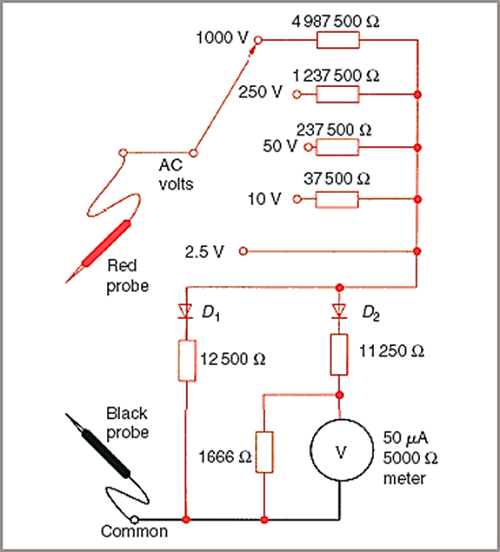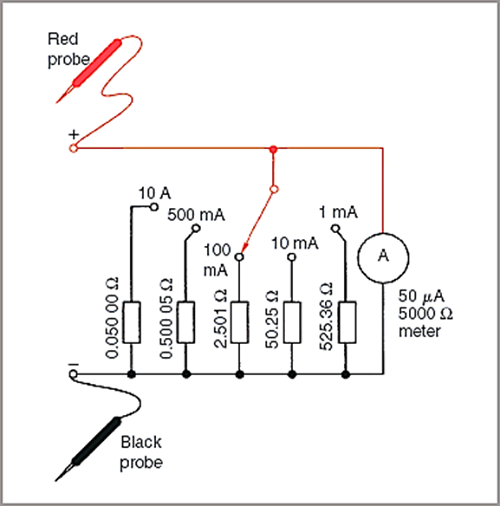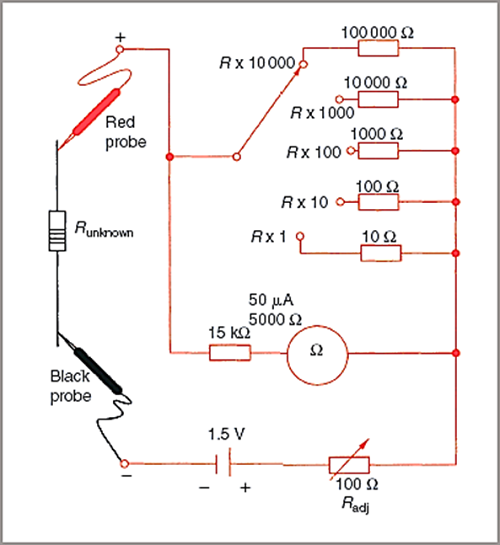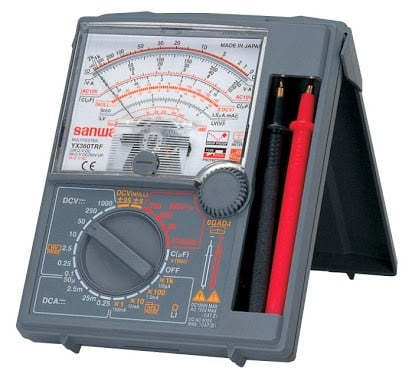Sometimes called a “volt-ohm meter” (a VOM), or an “ammeter, voltmeter, ohmmeter” (an AVO, which is also a registered trade name), an analog multimeter is a single meter with switching arrangements to connect it as either a voltmeter, ammeter or ohmmeter. The normal practice is to provide several ranges for each function.

The voltmeter section

Figure 1 overleaf shows a moving coil 50 μA meter with an internal resistance of 5 kΩ connected to a five-position switch. Each switch position connects a different value resistor in series (a multiplier) with the meter to provide a range of voltages.Figure 1: Simplified VOM schematic diagram. Source: Ahmed Faizan Ahmed

The moving-coil meter movement can read-only DC, so some means of rectifying AC must be provided.

The present trend is to provide half-wave rectification only, so it is imperative to have a second set of meter scales calibrated for half-wave-rectified alternating voltages. This type of circuit will also read DC voltages, so for accuracy, it is essential that the meter be set on the correct range and voltage. A circuit showing a meter circuit with five voltage ranges and half-wave rectification is shown in Figure 2. For comparison purposes, the same type of meter is used in both circuits.Figure 2: Multi-range AC voltmeter. Source: Ahmed Faizan Ahmed

The ammeter section

Figure 3 shows the same 50 μA meter connected to a five-position switch so that different values of shunt resistance can be connected in parallel with the meter. Again, it can operate on only DC because of the type of movement.Figure 3: Multi-range ammeter. Source: Ahmed Faizan Ahmed

Normal practice is to provide only DC operation for the current ranges. For AC, a special type of transformer must be included in the circuit. Called a current transformer, it is comparatively heavy, expensive and bulky. Multimeters are obtainable for measuring AC, but because of the expense involved are not in common use.

The ohmmeter section

Figure 4 shows the 50 μA meter connected in a series ohmmeter circuit. The circuit from Figure 1 has been modified slightly. The meter still includes a series of the current-limiting resistor, but meter shunts are incorporated into the switching arrangements. As the resistance range to be used increases in value, so does the value of the resistor shunting the meter.Figure 4: Typical ohmmeter circuit used in VOMs. Source: Ahmed Faizan Ahmed

Assuming that the meter is provided with only one resistance scale, a reading of 2 Ω while the meter is switched to the "R × 1" scale will be just that: 2 Ω. If the meter is switched to the "R × 100" scale, then when the scale indicates 2 Ω, the actual value will be 2 Ω 100 = 200 Ω.

The change in values of the shunt resistors has one important effect on the measuring current. On the lower resistance ranges, the current flowing through the unknown resistor is much higher in value. On the scale "multiplied by one" or "R × 1" range, for example, the measuring current is well more than 100 mA. On the "R × 10 000" range, the current through the resistor being measured is reduced to 15 μA plus the meter current, which the meter demands to be always a maximum of 50 μA.

The variation in measuring current has both advantages and disadvantages, depending on the circumstances in which the meter is being used. Trying to measure a low resistance semiconductor on the "R × 1" scale, for example, can destroy the device.

The complete multimeter

For a complete multimeter, all components are assembled in a container with a meter and batteries. For the units described in the three previous sections, the rotary switch would have a minimum of twenty positions. That is, five each for the DC volts, AC volts, DC current and resistance ranges. In this case, there would be a need for only two terminals — positive and negative, although the negative terminal is sometimes labeled "common."

High current ranges can be a cause for concern. The meter's components, such as shunt resistors and the rotary switch, must be capable of carrying the current, and alternative arrangements are sometimes made by using an extra shunt and terminal, appropriately labeled.

Manufacturers of multimeters make many different models, so individual instructions need to be supplied with each meter sold. Users of the meters are advised to read the manufacturer's instructions carefully until familiar with a particular meter.

The most obvious precaution to take with any meter is to ensure that it is set to the correct scale for the expected values to be measured. For example, to measure a domestic supply voltage, the meter needs to be set to a scale that can handle 240 V AC and at the same time have an upper scale reading in excess of this figure.

If the value of the voltage is not known, the meter should be set to the highest possible AC scale and only brought down to lower scales after each test until an adequate reading is obtained. It is always good practice to leave a meter on the highest AC voltage scale when not in use.

Under most conditions, an electrical worker need not consider the polarity of the ohmmeter terminals. It should be noted, however, that many meters reverse their polarities when switched to the resistance scales. That is, the red or positive terminal becomes the negative supply lead, and the black lead becomes the positive supply lead. This does not apply to all meters, so it is essential that the user check the characteristics of the instrument being used.

Modern multimeters are quite sensitive and often give a full-scale deflection with a current of microamperes. Because of this sensitivity, the resistance of the meter on the voltage ranges is so high that the loading on the circuit being measured can be ignored. Typically, a cheap multimeter on a 200 V DC range has a resistance of 20 000 Ω/V, so that on a 300 V full-scale deflection setting, the meter resistance between probes is 6 MΩ. On an AC range, it is around 10 000 Ω/V. Better meters have resistances on DC of 10 MΩ/V and 1 MΩ/V. Figure 5 shows a commercially available multimeter.Figure 5: Sanwa 460-ED multimeter. Source: Sanwa Multimeters

Range and function selection is with the aid of one and sometimes two rotary switches, usually electrically interlocked with each other.